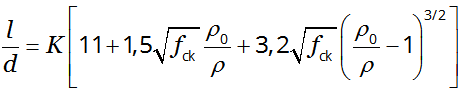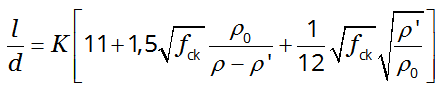For full functionality of this site it is necessary to enable JavaScript. Here are the instructions how to enable JavaScript in your web browser.

# Deflection control: the limit span/depth l/d for omitting calculations

## Eurocode 2 part 1-1: Design of concrete structures \$(document).ready(function () { var freeExample = \$("#freeExample").length; \$("#mainMenu li#nav-appCate, #mainMenu li#nav-appEC211").addClass("active"); var proUser = false === true || false === true || freeExample !== 0; if (!proUser) { \$('#Input input').keyup(function(){ \$('#AlertSubscribe').modal(); }); \$('#Input select').change(function(){ \$('#AlertSubscribe').modal(); }); }; var tempInput = \$("#tempInput").text(); if (tempInput != "") { \$("#Input").parent("form").deserialize(tempInput); if (proUser) { \$("#acceptBtn").trigger("click"); } }; });  7.4.2 (2)

If reinforced concrete beams or slabs in buildings are dimensioned so that they comply with the limits of span to depth ratio given in this application, their deflections may be considered as not exceeding the limits set out in § 7.4.1 (4) and § 7.4.1 (5). The limiting span/depth ratio may be estimated using Expressions (7.16.a) and (7.16.b), and multiplying this by correction factors to allow for the type of reinforcement used and other variables.if ρ ≤ ρ0 (7.16.a)if ρ > ρ0 (7.16.b)

where:

l/d
is the limit span/depth
K
is the factor to take into account the different structural systems, see § 7.4.2 (2)
ρ0
is the reference reinforcement ratio = 10-3 fck
ρ
is the required tension reinforcement ratio at mid-span to resist the moment due to the design loads (at support for cantilevers)
ρ'
is the required compression reinforcement ratio at mid-span to resist the moment due to design loads (at support for cantilevers)
fck
is the characteristic compressive strength of concrete, in [MPa], see Table 3.1.
• Expressions (7.16.a) and (7.16.b) are used while the steel stress, under the appropriate design load at SLS at a cracked section at the mid-span of a beam or slab or at the support of a cantilever, is 310 MPa, (corresponding roughly to fyk = 500 MPa). For other stress levels, the values of l/d given by (7.16) should be multipe by 310/σs. This factor will normally be conservative to assume to follow the Expression (7.17).
• For flanged sections where the ratio of the flange breadth to the rib breadth exceeds 3, the values of l/d given by (7.16) should be multiplied by 0,8.
• For beams and slabs, other than flat slabs, with spans exceeding 7 m, which support partitions liable to be damaged by excessive deflections, the values of l/d given by (7.16) should be multiplied by 7/leff (leff in [m], cf. 5.3.2.2 (1)).
• For flat slabs where the greater span exceeds 8,5 m, and which support partitions liable to be damaged by excessive deflections, the values of l/d given by (7.16) should be multiplied by 8,5/leff (leff in [m], cf. 5.3.2.2 (1)).

This application calculates the limit span/depth l/d from your inputs.

Input
MPa

Output
ρ0
the limit span/depth l/d